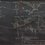# Proposal for Brilliant Polymath Project

Classify all (rational) $q$ such that, if $e^q$ has the continued fraction expansion $[a_0;a_1,a_2,\ldots]$, $\{ a_{mn+c} \}_{n=0}^\infty$ is an arithmetic progression for some $m$ and every $c>0$.

(Conjectured: All $q$ rational have this property.)

As examples, observe the following expansions:

\begin{aligned} q = 1, & e^q = [2; 1, 2, 1, 1, 4, 1, 1, 6, 1, 1, 8, 1, 1, 10, 1, \ldots], & m_{\min} = 3 \\ q = 2, & e^q = [7; 2, 1, 1, 3, 18, 5, 1, 1, 6, 30, 8, 1, 1, 9, 42, 11, 1, 1, 12, 54, \ldots], & m_{\min} = 5 \\ q = 1/2, & e^q = [1; 1, 1, 1, 5, 1, 1, 9, 1, 1, 13, 1, 1, 17, 1, 1, \ldots], & m_{\min} = 3 \\ \end{aligned}

Known facts:

$e^z = \cfrac{1}{1 - \cfrac{z}{1 + z - \cfrac{z}{2 + z - \cfrac{2z}{3 + z - \cfrac{3z}{4 + z - \ddots}}}}}$

(Due to Euler.)Note by Jake Lai
5 years, 5 months ago

This discussion board is a place to discuss our Daily Challenges and the math and science related to those challenges. Explanations are more than just a solution — they should explain the steps and thinking strategies that you used to obtain the solution. Comments should further the discussion of math and science.

When posting on Brilliant:

• Use the emojis to react to an explanation, whether you're congratulating a job well done , or just really confused .
• Ask specific questions about the challenge or the steps in somebody's explanation. Well-posed questions can add a lot to the discussion, but posting "I don't understand!" doesn't help anyone.
• Try to contribute something new to the discussion, whether it is an extension, generalization or other idea related to the challenge.

MarkdownAppears as
*italics* or _italics_ italics
**bold** or __bold__ bold
- bulleted- list
• bulleted
• list
1. numbered2. list
1. numbered
2. list
Note: you must add a full line of space before and after lists for them to show up correctly
paragraph 1paragraph 2

paragraph 1

paragraph 2

[example link](https://brilliant.org)example link
> This is a quote
This is a quote
    # I indented these lines
# 4 spaces, and now they show
# up as a code block.

print "hello world"
# I indented these lines
# 4 spaces, and now they show
# up as a code block.

print "hello world"
MathAppears as
Remember to wrap math in $$ ... $$ or $ ... $ to ensure proper formatting.
2 \times 3 $2 \times 3$
2^{34} $2^{34}$
a_{i-1} $a_{i-1}$
\frac{2}{3} $\frac{2}{3}$
\sqrt{2} $\sqrt{2}$
\sum_{i=1}^3 $\sum_{i=1}^3$
\sin \theta $\sin \theta$
\boxed{123} $\boxed{123}$

Sort by:

How did you come across such observation?

- 5 years, 5 months ago

I noted the regularity of e's expansion in a YouTube lecture and thought that, since e is intimately linked with exponentiation, $e^x$ might display similar regularity as well.

- 5 years, 5 months ago

A good place to start might be to prove this for $q = \frac{1}{x}$ ($x\in \mathbb{N}$), which Euler himself actually proved.

Staff - 5 years, 5 months ago

Where is this result found? It would be great to look at the proof for insight :)

- 5 years, 5 months ago

Here is an English translation of Euler's paper.

Staff - 5 years, 5 months ago

Staff - 5 years, 5 months ago

I have not, sadly. Been too busy with too much :<

- 5 years, 5 months ago